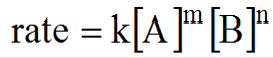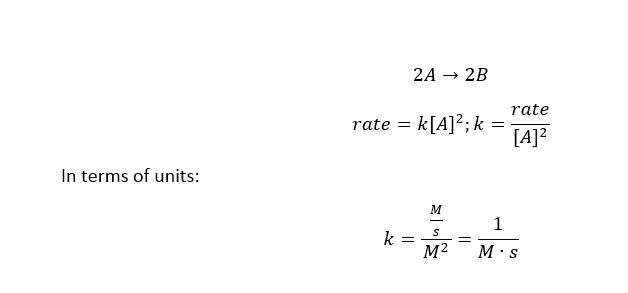# Order and molecularity of a reaction

Chemical Kinetics: Order and molecularity of a reaction

Order

The rate law shows an expression or equation which associates the rate of reaction to the concentrations of reactants at a constant temperature. The rate of a reaction depends on concentration while the rate constant is affected by temperature and the presence of a catalyst.

The order of a reaction is the power or exponent of the concentration for a given reactant denoted in the rate law. It indicates the degree to which the rate depends on the concentration of that reactant. Accordingly, the sum of the powers of the concentrations is considered the overall order for the reaction. In a 1st order reaction, if the concentration doubles, the rate doubles. In a 2nd order reaction, if the concentration doubles, the rate quadruples. Likewise, in a 3rd order reaction, if the concentration doubles, the rate increases by the power of 9.

Consider the reaction A+B –> ProductsWhere, k=rate constant and m, n are the order of the reaction which can either be 0, fractional or negative.

Likewise, for the reaction:

R1 + R2 + R3 –> Products

Rate = k[R1]x[R2]y[R3]z

Where k = rate constant; x, y, and z are the rate orders which are determined experimentally.

For example

Rate = k[NH4+][NO2]; where NH4+ = 1st order and NO2 = 1st order. Therefore, the overall reaction order = 2nd order

The units for the rate constant depend on the overall reaction order of the rate law.

For exampleMolecularity

The molecularity of a process denotes the number of any molecules that are involved in the process. It is the number of molecules present in the elementary step of a reaction. Any process that occurs in a single step is called elementary. There are several types of elementary steps:

Unimolecular: one molecule in the elementary step, A–>P

Bimolecular: two molecules in the elementary step, and A+A –> P; A+B –> P

Termolecular: three molecules in the elementary step, A+B+C –> P; 2A+B –> P

Higher molecularities are rare because of the small statistical probability that four or more molecules would all collide at the same instant.

An elementary reaction is considered as the actual molecular event. This means that the rate of an elementary reaction is proportional to the concentration of each reactant molecule. Hence, we can express the rate law directly from an elementary reaction. So for an elementary reaction, the coefficient of each reactant becomes the power to which it is raised in the rate law for that reaction. Note that many chemical reactions occur in multiple steps and it is, therefore, impossible to predict the rate law based solely on the overall reaction.

Consider the following example where the elementary steps in the reaction of NO2 and CO are shown.

NO2(g) + CO(g) → NO(g) + CO2(g)

Step-1: NO2 + NO2 NO3 + NO

Step-2: NO3+ CO NO2 + CO2

Overall: NO2 + CO NO + CO2

The experimental rate law is Rate = k[NO2]2 . It implies that the above reaction is second-order w.r.t. NO2 , but is zero-order in [CO].The sum of the elementary steps must give the overall balanced equation and the mechanism must correspond with the experimentally determined rate law for the reaction.Fig1: Elementary steps and rate laws.